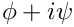oomph::LAPACK_QZ Class Reference

Class for the LAPACK eigensolver. More...

#include <eigen_solver.h>Inheritance diagram for oomph::LAPACK_QZ:

## Public Member Functions

LAPACK_QZ ()
Empty constructor. More...

LAPACK_QZ (const LAPACK_QZ &)
Empty copy constructor. More...

virtual ~LAPACK_QZ ()
Empty desctructor. More...

void solve_eigenproblem (Problem *const &problem_pt, const int &n_eval, Vector< std::complex< double > > &eigenvalue, Vector< DoubleVector > &eigenvector)
Solve the eigen problem. More...

void find_eigenvalues (const ComplexMatrixBase &A, const ComplexMatrixBase &M, Vector< std::complex< double > > &eigenvalue, Vector< Vector< std::complex< double > > > &eigenvector)
Find the eigenvalues of a generalised eigenvalue problem specified by. More...

void get_eigenvalues_right_of_shift ()
Set the desired eigenvalues to be right of the shift Dummy at the moment. More...Public Member Functions inherited from oomph::EigenSolver
EigenSolver ()
Empty constructor. More...

EigenSolver (const EigenSolver &)
Empty copy constructor. More...

virtual ~EigenSolver ()
Empty destructor. More...

void set_shift (const double &shift_value)
Set the value of the shift. More...

const double & get_shift () const
Return the value of the shift (const version) More...Public Member Functions inherited from oomph::DistributableLinearAlgebraObject
DistributableLinearAlgebraObject ()
Default constructor - create a distribution. More...

DistributableLinearAlgebraObject (const DistributableLinearAlgebraObject &matrix)=delete
Broken copy constructor. More...

void operator= (const DistributableLinearAlgebraObject &)=delete
Broken assignment operator. More...

virtual ~DistributableLinearAlgebraObject ()
Destructor. More...

unsigned nrow () const
access function to the number of global rows. More...

unsigned nrow_local () const
access function for the num of local rows on this processor. More...

unsigned nrow_local (const unsigned &p) const
access function for the num of local rows on this processor. More...

unsigned first_row () const
access function for the first row on this processor More...

unsigned first_row (const unsigned &p) const
access function for the first row on this processor More...

bool distributed () const
distribution is serial or distributed More...

bool distribution_built () const
if the communicator_pt is null then the distribution is not setup then false is returned, otherwise return true More...

void build_distribution (const LinearAlgebraDistribution *const dist_pt)
setup the distribution of this distributable linear algebra object More...

setup the distribution of this distributable linear algebra object More...Protected Member Functions inherited from oomph::DistributableLinearAlgebraObject
void clear_distribution ()
clear the distribution of this distributable linear algebra object More...Protected Attributes inherited from oomph::EigenSolver
double Sigma_real
Double value that represents the real part of the shift in shifted eigensolvers. More...

## Detailed Description

Class for the LAPACK eigensolver.

Definition at line 223 of file eigen_solver.h.

## ◆ LAPACK_QZ() [1/2]

 oomph::LAPACK_QZ::LAPACK_QZ ( )
inline

Empty constructor.

Definition at line 227 of file eigen_solver.h.

## ◆ LAPACK_QZ() [2/2]

 oomph::LAPACK_QZ::LAPACK_QZ ( const LAPACK_QZ & )
inline

Empty copy constructor.

Definition at line 230 of file eigen_solver.h.

## ◆ ~LAPACK_QZ()

 virtual oomph::LAPACK_QZ::~LAPACK_QZ ( )
inlinevirtual

Empty desctructor.

Definition at line 233 of file eigen_solver.h.

## ◆ find_eigenvalues()

 void oomph::LAPACK_QZ::find_eigenvalues ( const ComplexMatrixBase & A, const ComplexMatrixBase & M, Vector< std::complex< double > > & eigenvalue, Vector< Vector< std::complex< double > > > & eigenvector )

Find the eigenvalues of a generalised eigenvalue problem specified by.

Use LAPACK to solve a complex eigen problem specified by the given matrices.

Definition at line 707 of file eigen_solver.cc.

References i, and oomph::ComplexMatrixBase::nrow().

## ◆ get_eigenvalues_right_of_shift()

 void oomph::LAPACK_QZ::get_eigenvalues_right_of_shift ( )
inline

Set the desired eigenvalues to be right of the shift Dummy at the moment.

Definition at line 250 of file eigen_solver.h.

## ◆ solve_eigenproblem()

 void oomph::LAPACK_QZ::solve_eigenproblem ( Problem *const & problem_pt, const int & n_eval, Vector< std::complex< double > > & eigenvalue, Vector< DoubleVector > & eigenvector )
virtual

Solve the eigen problem.

Use LAPACK to solve an eigen problem that is assembled by elements in a mesh in a Problem object.

Implements oomph::EigenSolver.

Definition at line 540 of file eigen_solver.cc.

The documentation for this class was generated from the following files: